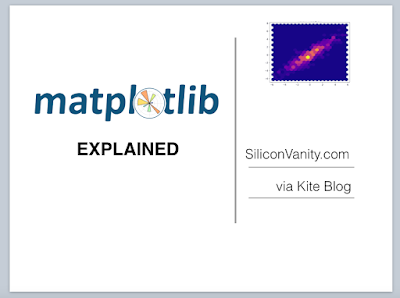Sunday, November 12, 2017

What is Tensorflow? - Tensorflow for Dummies Google Tensorflow 101

What is Tensorflow

Tensorflow is a deep learning framework, and deep learning is a hot field of machine learning. It's like like rails is a framework for web applications and bootstrap is a framework for front end development.

More generally, Tensorflow is built for large scale numerical computation. Deep learning is one of its capabilities.

You can scale your machine learning code, with Google in the cloud by employing more than one core. Even use GPU and parallel processing.

Tensorflow computes gradients, a non trivial calculation, fast.

TF provides a library of machine learning APIs, models, scoring metrics, optimizer for machine learning. It also provide mathematical computation libraries and functions that support high dimension matrix calculation, manipulation for linear algebra.

Get a taste of Tensorflow for beginners here: https://www.tensorflow.org/get_started/mnist/beginners
Get a taste of Tensorflow for experts here: https://www.tensorflow.org/get_started/mnist/pros
Google Cloud app engine will soon offer Cloud ML